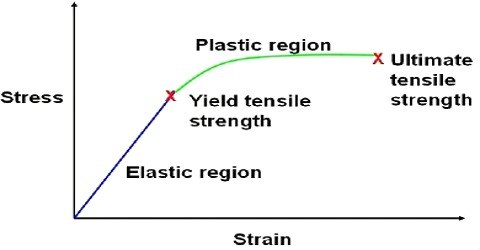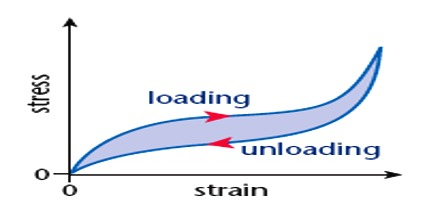# What is Strain?

Strain: The change produced in the dimensions of a body under a system forces or couples in equilibrium is called strain. This strain can be linear, shear or volume according to the change takes place in length, shape and volume of the body. The fact that strain means the relative change in shape or size implies that it is dimensionless and has no units.Normal stress on a body causes change in length or volume and tangential stress produces the change in shape of the body. The ratio of change produced in the dimensions of a body by a system of forces or couples, in equilibrium, to its original dimensions is called strain.

Strain = [Change in dimensions / original dimensions]

Explanation: Let the initial dimension of a body = x

and the dimension after the applied force = y

So, change of dimension = x ≈ y

then, change per unit dimension i.e., strain = (x ≈ y) / x

Types: There are three types of strain. e.g.,

(i) Longitudinal strain (ii) Shearing strain and (iii) Volume strain.

Unit and dimension: It is a ratio of two similar quantities. So it has no unit or dimension.